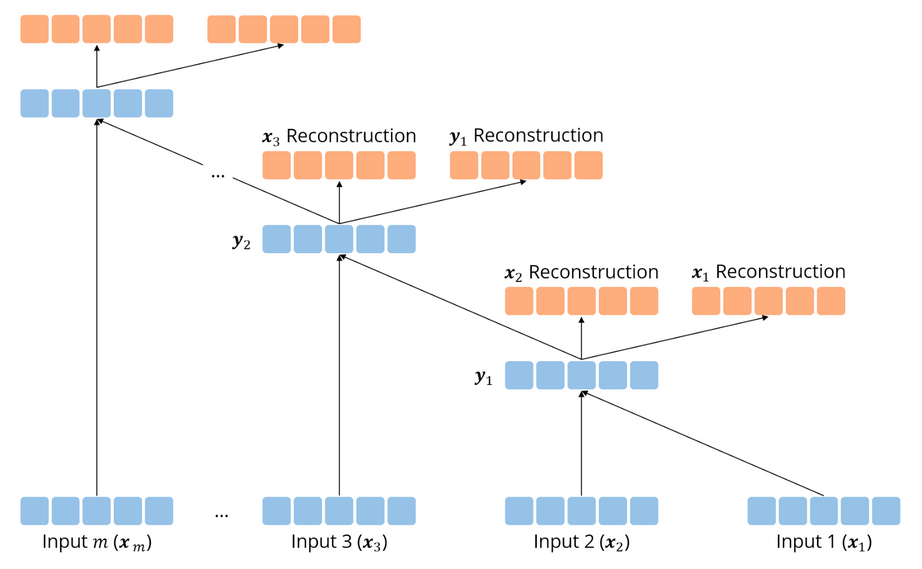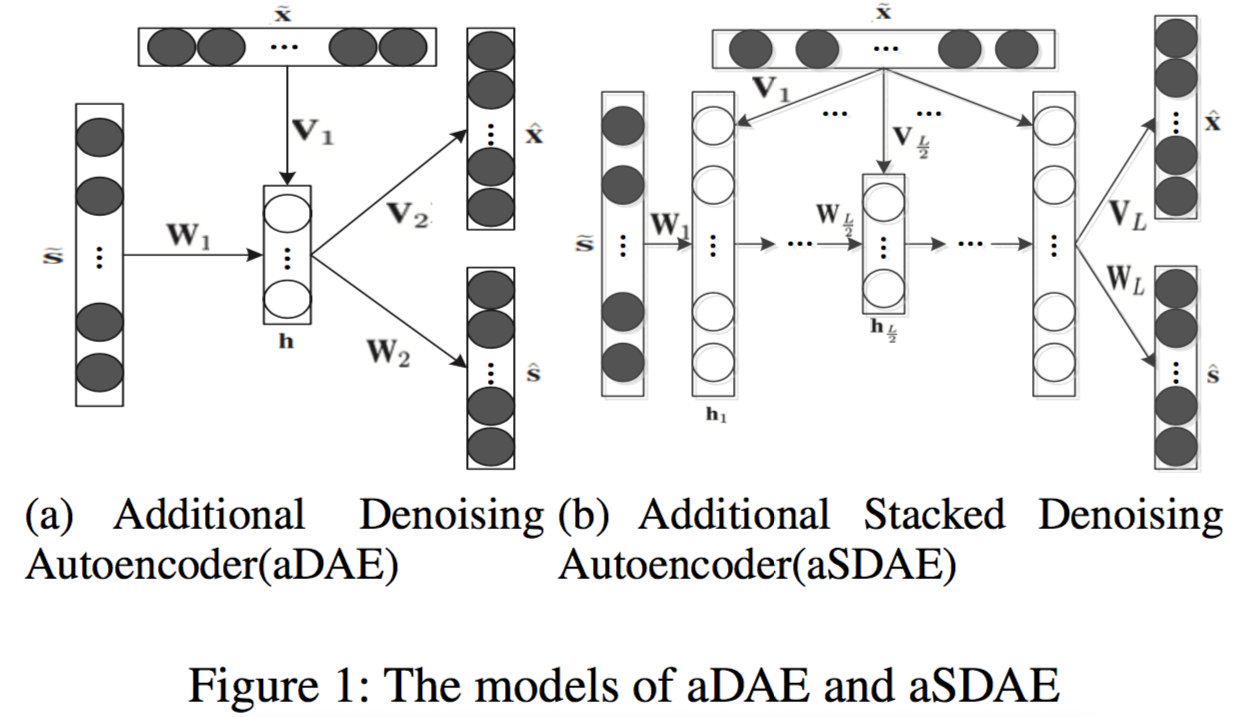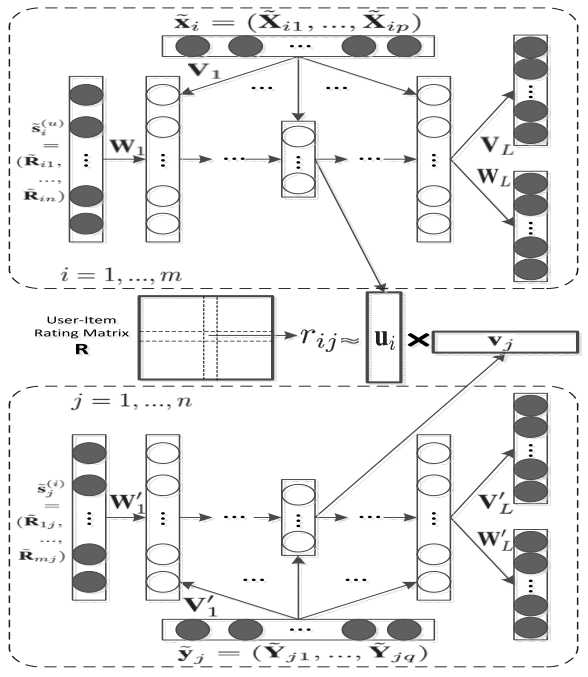• 递归自动编码器（Recursive Autoencoder）

“自动编码器一览”系列：

## 递归自动编码器### 传统递归自动编码器

$$p=f\left(W^{(1)}\left[c_{1} ; c_{2}\right]+b^{(1)}\right),$$

$$\left[c_{1}^{\prime} ; c_{2}^{\prime}\right]=W^{(2)} p+b^{(2)}$$

$$E_{r e c}\left(\left[c_{1} ; c_{2}\right]\right)=\frac{1}{2}\left|\left[c_{1} ; c_{2}\right]-\left[c_{1}^{\prime} ; c_{2}^{\prime}\right]\right|^{2}$$

### 用于预测结构的无监督递归自动编码器

$$E_{r e c}\left(\left[c_{1} ; c_{2}\right] ; \theta\right)= \frac{n_{1}}{n_{1}+n_{2}}\left|c_{1}-c_{1}^{\prime}\right|^{2}+\frac{n_{2}}{n_{1}+n_{2}}| | c_{2}-c_{2}^{\prime}| |^{2}$$

### 半监督递归自动编码器$$h_{l}=g\left(W_{l} h_{l-1}+V_{l} \tilde{x}+b_{l}\right),$$

\begin{aligned} \hat &=f\left({W}_{L} {h}_{L}+{b}_{\hat{s}}\right) \\ \hat &=f\left({V}_{L} {h}_{L}+{b}_{\hat{x}}\right) \end{aligned}

$$L = \alpha|{s}-\hat|_{F}^{2}+(1-\alpha)|{x}-\hat|_{F}^{2} + \lambda\left(\sum_{l}\left|{W}_{l}\right|_{F}^{2}+\left|{V}_{l}\right|_{F}^{2}\right),$$

aSDAE 本身就介绍完了。在论文  中，aSDAE 学习用户和商品对应的隐向量矩阵，并将用户的基本个人信息、用户画像信息、物品的基本信息等附加信息引入，从而解决协同过滤中的稀疏性与冷启动问题。这些和推荐系统相关的背景知识就不多讨论了，有兴趣的同学可以看参考资料 。## More Autoencoder

1. 为什么要最小化重构误差？有没有其他做法？
2. 怎么让自动编码器学习到的表征更具可解释性？

## 参考资料

•  Richard Socher, Jeffrey Pennington, Eric H.Huang, Andrew Y.Ng, Christopher D.Manning. “Semi-Supervised Recursive Autoencoders for Predicting Sentiment Distributions”, EMNLP 2011
•  Xin Dong, Lei Yu, Zhonghuo Wu, Yuxia Sun, Lingfeng Yuan, Fangxi Zhang. “A Hybrid Collaborative Filtering Model with Deep Structure for Recommender Systems”, AAAI 2017
•  推荐系统中基于深度学习的混合协同过滤模型. https://zhuanlan.zhihu.com/p/25234865